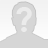### How to call a transaction every n iterations

In my user path's Actions i have several transactions. some of them i don't want to call every iteration but e.g. every 10th iteration. Usually i'd create a variable e.g. \${numIter} and increase this variable by 1 each iteration. In the user path's action I'd insert an if-then-else. and create if \${numIter}%10 == 0 condition. But actually i can't find a modulo operator in the Operator List of the "Condition" Element.

Are there other approaches?

Markus G.#### Markus G.

Level
0
17 / 100
points

the action_counter variable would tell you the current iteration. You could then pass this to some JavaScript (see actions section bottom left) that calculates the remainder and sets a variable. You can then use this in your Neoload IF statement "\${remainder} equals 0"

Did you find this useful ?

(0)

(1)

100%

100% of users found this answer useful